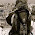## Sunday, April 13, 2014

### Factorization in coNP- in other domains?

I had on an exam in my grad complexity course to show that the following set is in coNP

FACT = { (n,m) : there is a factor y of n with 2 \le y \le m }

The answer I was looking for was to write FACTbar (the complement) as

FACTbar = { (n,m) | (\exists p_1,...,p_L) where L \le log n
for all i \le L we have m < p_i \le n and p_i is prime (the p_i are not necc distinct)
n =p_1 p_2 ... p_L
}
INTUITION: Find the unique factorization and note that the none of the primes are < m
To prove this work you seem to need to use the Unique Factorization theorem and you need
that PRIMES is in NP (the fact that its in P does not help).

A student who I will call Jesse (since that is his name) didn't think to complement the set  so instead he wrote the following CORRECT answer

FACT = { (n,m) | n is NOT PRIME and forall p_1,p_2,...,p_L  where 2\le L\le log n
for all i \le L,  m< p_i \le n-1 , (p_i prime but not necc distinct).
n \ne p_1 p_2 ... p_L
}
(I doubt this proof that FACT is in coNP is new.)
INTUITION: show that all possible ways to multiply together numbers larger than m do not yield n,
hence n must have a factor \le m.

Here is what strikes me- Jesse's proof does not seem to use Unique Factorization.  Hence it can be used in other domains(?). Even those that do not have Unique Factorization (e.g. Z[\sqrt{-5}]. Let D= Z[\alpha_1,...,\alpha_k] where the alpha_i are algebraic. If n\in D then let N(n) be the absolute value of the sum of the coefficients (we might want to use the product of n with all of its conjugates instead, but lets not for now).

FACT = { (n,m) : n\in D, m\in NATURALS, there is a factor y in D of n with 2 \le N(y) \le m}

Is this in NP? Not obvious (to me) --- how many such y's are there.

Is this the set we care about? That is, if we knew this set is in P would factoring be in P? Not obv (to me).

I suspect FACT is in NP, though perhaps with a diff definition of N( ). What about FACTbar?
I think Jesse's approach works there, though might need  diff bound then log L.

I am (CLEARLY) not an expert here and I suspect a lot of this is known, so my real point is
that a students diff answer then you had in mind can be inspiring. And in fact I am inspired to
read Factorization: Unique and Otherwise by Weintraub which is one of many books I've been
meaning to read for a while.

1.The various definitions of FACT and FACT-bar seem have the form { (n,m) | }. I'm pretty good at figuring out what you mean, but I can't tell what Jesse means (Are there more typos in there? Did you really mean it when you said "n \ne p_1 p_2 ... p_L"?)

It would also be helpful to get an inline LaTeX parser for the blog!

2.Has Jesse's proof been garbled? If I am understanding it right, it says FACT is the set of (n,m) such that every factorization of n contains a factor between 2 and m, which is not correct.

3.YES, I garbled things and also my editor garbled things. But its fixed now. I hope.

4.Maybe I'm confused, but is Jesse's proof really correct? A pair (n=64, m=6) belongs to FACT (2 is a factor). But in this definition, for L=2, p1=p2=8 we have that it does not belong.

1.It looks like you're right.
Couldn't we add in the condition that each p_i is prime? PRIMES is clearly in co-NP: for all q, r, q*r=p -> q=1 or r=1.

5.maybe someone should answer malcin's concern ?

1.Malcin is correct, and Andy is correct, so I used Andy's correction.

6.Here http://npcomplete-001-site1.myasp.net/ resolved Exact cover ploblem, on line solver aviable

7.What about adding MathJax to the site? (one line of code :-)